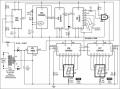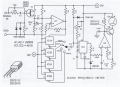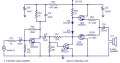# I am a beginner in electronics and I have two questions

#### wtmma

Joined Dec 21, 2012
11
Hello,
I am a beginner in electronics and I have two questions I hope you can help me.

1- I have studied and learned Ohm law, circuit analysis, circuit reduction and Kirchhoff's laws. I want to know whether circuit analysis methods and Kirchhoff's laws are used in detecting faults, or in the circuit design stage, and how this is done.

2- In all the content I have studied so far, all the problems and exercises are applied to circuits made up of only resistors. I want to know that in the case of real circuits, which contain other electronic components, how they are dealt with in terms of applying Ohm's law or Kirchhoff's laws. What I mean is that in circuits that contain only resistors, we can do all the calculations because the values of the resistors are known to us, but in circuits that contain other components with resistors, how can we know the value of the resistance of those components in order to perform the calculations.

3- In the design phase of any new circuit, we first put all the components of the circuit and connect them with each other, and then calculate the voltages and currents needed for those components? Or do we put the circuit components one by one and calculate the voltages and currents for each component?

Many thanks for Help..

Walid

#### Ian0

Joined Aug 7, 2020
6,707
Networks of more than two resistors don’t show up very often in circuit design.
The design process consists mainly of connecting together blocks of known functionality
What were you thinking of designing?.

#### MrChips

Joined Oct 2, 2009
27,681
Hello Walid. These are all very valid questions and there is no easy answer.

I would suggest that we examine three types of circuits:
1) circuits with discrete components, R, C, L, and semiconductor devices,
2) circuits with analog ICs,
3) circuits with digital ICs.

Starting with (3), there is usually little or no calculations required. With digital gates we use boolean algebra to analyze combinational circuits. For any part of the circuit with discrete components analysis (1) will apply.With analog or mixed signal designs, ICs are considered "black boxes". Component values are usually what the IC manufacturer recommends. Again we rarely need to perform a numerical analysis. With experience, we learn circuit topology, i.e. typical configuration of circuits, for example, op-amps, active filters, timing circuits. Known formulas are used to calculate the performance of the circuit with the given components.Finally with discrete components (1), R, C, L circuits can be analyzed with known formulas. When you add non-linear devices such as diodes and transistors you cannot use Ohm's Law alone to analyze the circuit. Again, with experience you will become acquainted with circuit topology, how the circuit behaves as a whole, and how to calculate currents and voltages using known rules and approximations. For most part, you can isolate specific parts of a complex circuit and determine the current, voltage or AC response of the circuit, for example, RC filters, timing circuits, resistor and diode circuits, etc.I have intentionally shown relatively complex circuit diagrams as examples. It would be a nightmare attempting a calculate every current and voltage on each node and component. Instead, we learn the function of each component in the circuit. We can have an estimate or expected values of current and voltage of a specific section of the circuit.

So to directly answer your question, you learn how to analyze circuits with just two components in a circuit. Then you start building and using more complex circuits. In time you will acquire enough experience to understand and create more complex circuits on your own.

How children acquire natural language is a good analogy. Children learn single words, one at a time, such as mama, dog, water. Then they learn word combinations, my mama, dog run, cold water. Finally, they learn how to create complete sentences.

I hope this helps.

•Sinus23

#### wtmma

Joined Dec 21, 2012
11
Networks of more than two resistors don’t show up very often in circuit design.
The design process consists mainly of connecting together blocks of known functionality
What were you thinking of designing?.
Hallo Ian0,
I am not yet in grade of designing a circuit, but I wanted to now the way of doing that. many thanks for ur answer.

#### Ian0

Joined Aug 7, 2020
6,707
Hallo Ian0,
I am not yet in grade of designing a circuit, but I wanted to now the way of doing that. many thanks for ur answer.
Often it is easier to illustrate how it is done if you have a project in mind, rather that trying to explain it for an arbitrary circuit.

•wtmma

#### wtmma

Joined Dec 21, 2012
11
Hello Walid. These are all very valid questions and there is no easy answer.

I would suggest that we examine three types of circuits:
1) circuits with discrete components, R, C, L, and semiconductor devices,
2) circuits with analog ICs,
3) circuits with digital ICs.

Starting with (3), there is usually little or no calculations required. With digital gates we use boolean algebra to analyze combinational circuits. For any part of the circuit with discrete components analysis (1) will apply.

View attachment 278650

With analog or mixed signal designs, ICs are considered "black boxes". Component values are usually what the IC manufacturer recommends. Again we rarely need to perform a numerical analysis. With experience, we learn circuit topology, i.e. typical configuration of circuits, for example, op-amps, active filters, timing circuits. Known formulas are used to calculate the performance of the circuit with the given components.

View attachment 278651

Finally with discrete components (1), R, C, L circuits can be analyzed with known formulas. When you add non-linear devices such as diodes and transistors you cannot use Ohm's Law alone to analyze the circuit. Again, with experience you will become acquainted with circuit topology, how the circuit behaves as a whole, and how to calculate currents and voltages using known rules and approximations. For most part, you can isolate specific parts of a complex circuit and determine the current, voltage or AC response of the circuit, for example, RC filters, timing circuits, resistor and diode circuits, etc.

View attachment 278652

I have intentionally shown relatively complex circuit diagrams as examples. It would be a nightmare attempting a calculate every current and voltage on each node and component. Instead, we learn the function of each component in the circuit. We can have an estimate or expected values of current and voltage of a specific section of the circuit.

So to directly answer your question, you learn how to analyze circuits with just two components in a circuit. Then you start building and using more complex circuits. In time you will acquire enough experience to understand and create more complex circuits on your own.

How children acquire natural language is a good analogy. Children learn single words, one at a time, such as mama, dog, water. Then they learn word combinations, my mama, dog run, cold water. Finally, they learn how to create complete sentences.

I hope this helps.

#### Sensacell

Joined Jun 19, 2012
3,098
While calculations are essential, I feel this is over-emphasized by most electronics teaching.

I often hear beginners say "how do I calculate these circuit values" when there is not enough information or context to calculate anything.
This is because students get beaten to death with too much calculating, and not enough general thinking.

Circuit design starts with clearly defining objectives, then we explore circuit topologies that 'might' work to solve the problems. This is an iterative and creative process, not a rote exercise or recipe.
Once you have a potentially workable circuit topology, you can then begin to analyze the circuit by calculating values to illuminate the good and bad of the circuit.
You go round and round with this - until an acceptable cost/size/performance/ compromise is realized.

So study more circuit topology and gain a conceptual understanding of things, not only is this way more fun, but it makes the calculation part meaningful - instead of just hateful drudgery.

That's how things REALLY get designed, this is what nobody teaches students.

#### LvW

Joined Jun 13, 2013
1,586
Yes - I fully agree to Sensacells contribution and support all of his recommendations.
In addition , before starting such iterative design process I strongly recommend to understand the working principles and the main properties of electronic parts:
* Resistors
* Capacitors and inductors (coils) with frequency-dependent characteristics (phase shift!)
* pn junctions (Diodes) and its voltage-current relations
* Bipolar transistors (voltage-current relations)
* FET`s (voltage-current relations)

•Sensacell and wtmma

#### wtmma

Joined Dec 21, 2012
11
While calculations are essential, I feel this is over-emphasized by most electronics teaching.

I often hear beginners say "how do I calculate these circuit values" when there is not enough information or context to calculate anything.
This is because students get beaten to death with too much calculating, and not enough general thinking.

Circuit design starts with clearly defining objectives, then we explore circuit topologies that 'might' work to solve the problems. This is an iterative and creative process, not a rote exercise or recipe.
Once you have a potentially workable circuit topology, you can then begin to analyze the circuit by calculating values to illuminate the good and bad of the circuit.
You go round and round with this - until an acceptable cost/size/performance/ compromise is realized.

So study more circuit topology and gain a conceptual understanding of things, not only is this way more fun, but it makes the calculation part meaningful - instead of just hateful drudgery.

That's how things REALLY get designed, this is what nobody teaches students.
Thank you very much, you have touched the very important point in the learning process. I have made tons of resistors calculations, but what then. You have made the point very clear.
Pls. can you tell me about a possible learning path that I can follow. Because I am very confused about where to go first in this very long journey.

#### ericgibbs

Joined Jan 29, 2010
16,790
hi wtmm,
What electronic tools and equipment do you have available at this time.?
E

#### Ian0

Joined Aug 7, 2020
6,707
Thank you very much, you have touched the very important point in the learning process. I have made tons of resistors calculations, but what then. You have made the point very clear.
Pls. can you tell me about a possible learning path that I can follow. Because I am very confused about where to go first in this very long journey.
Which aspect of electronics are you most interested in?
Analogue? Digital? Radio? Audio? Power distribution? Mobile phone network? Microprocessors?

#### wtmma

Joined Dec 21, 2012
11
hi wtmm,
What electronic tools and equipment do you have available at this time.?
E
Hallo,
I am at the very beginning of learning. I have Breaboard and some components. I asked this questions because I want to now on what have i to concentrate

#### Sensacell

Joined Jun 19, 2012
3,098
These days, so much circuit design activity revolves around black box modules and digital solutions for everything.

I recommend reaching into the past, there are so many simple transistor circuits that can be built and tinkered with.
This knowledge transfers directly to the modern age, and will make you a far more competent engineer.

In my youth, I had this book called "the encyclopedia of electronic circuits" (Rudolph F Graf) it's just a huge compilation of circuits.
I would pour endlessly over the diagrams, trying to find patterns, and I did. The patterns you find represent small chunks of topography that you will find repeated everywhere in electronics.
After a while, you will be able to recognize the patterns and be able to associate them with the useful functions they perform.

Then I got my hands on tons of parts and played around with them until I had a basic, intuitive understanding of their behaviors and properties.

Then combining the understanding of the parts with the circuit topographies I learned, I began to understand how I could make what I wanted to happen - happen.

Calculations serve this process, not the other way around. Focus on understanding what's going on, rather than fixating on exactly how much is going on.
Once you understand how circuits function, you begin to understand that for many things, the exact number is actually meaningless, it's a compromise that works.
But of course, there are situations where it's essential to calculate the exact value of something- wisdom is knowing the difference.

My favorite example: Calculate the value of a pullup resistor in a digital circuit. Yes, math helps you to see the edges of the problem, but cannot provide an 'exact' answer.

Last edited:
•panic mode

#### bidrohini

Joined Jul 29, 2022
96
Hello,
I am a beginner in electronics and I have two questions I hope you can help me.

1- I have studied and learned Ohm law, circuit analysis, circuit reduction and Kirchhoff's laws. I want to know whether circuit analysis methods and Kirchhoff's laws are used in detecting faults, or in the circuit design stage, and how this is done.

2- In all the content I have studied so far, all the problems and exercises are applied to circuits made up of only resistors. I want to know that in the case of real circuits, which contain other electronic components, how they are dealt with in terms of applying Ohm's law or Kirchhoff's laws. What I mean is that in circuits that contain only resistors, we can do all the calculations because the values of the resistors are known to us, but in circuits that contain other components with resistors, how can we know the value of the resistance of those components in order to perform the calculations.

3- In the design phase of any new circuit, we first put all the components of the circuit and connect them with each other, and then calculate the voltages and currents needed for those components? Or do we put the circuit components one by one and calculate the voltages and currents for each component?

Many thanks for Help..

Walid
Real-life fault analysis is taught in the 'Power system analysis' course. We did it in the fourth year of Electrical Engineering.
Inductors and Capacitors are different from resistors. They have different equations of voltage and current. If you are interested, you can study inductor and capacitor I-V equations.

Datasheets are available for all the components. By reading datasheets, we can determine the required voltages and currents of each component. Thus we can design our circuits knowing the total power consumptions in prior.

•wtmma

#### wtmma

Joined Dec 21, 2012
11

#### Audioguru again

Joined Oct 21, 2019
5,416
A horrible schematic was made by students on the other side of the world and was in post #3 in this thread.
The schematic has errors and most circuits on that website have similar errors.
It is a "4 transistors audio amplifier" that produces a very distorted output power of almost nothing. I simulated its output amplifier:

#### MrChips

Joined Oct 2, 2009
27,681
@Audioguru again With due respect of your expert knowledge on audio amplifier circuit design, the sample circuits posted were not intended to be examples of properly functioning circuits. The purpose of the circuits was to introduce the concept of various circuit topology and how we learn to analyze circuits by looking at the function of individual components that comprise the circuit.

•ericgibbs